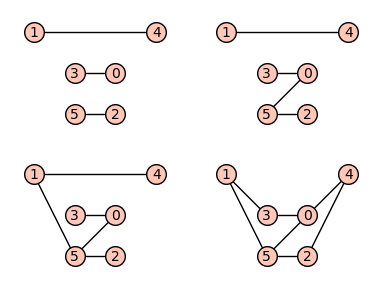# Graphs on 6 vertices with eigenvalue conditionAnonymous

Consider the class of all possible connected simple graphs on $6$ vertices. Now from this collection, can we find those graphs (if there exists any) satisfying the following property: Suppose that $A$ denotes the usual $(0,1)$ adjacency matrix of a graph. Now can we find those graphs explicitly (if there is any) on $6$ vertices such that if $\lambda$ is an eigenvalue of $A$, then $\dfrac{1}{\lambda}$ and $-\dfrac{1}{\lambda}$ both are eigenvalues. Simply the question can be said as: Characterize all possible simple connected graphs on $6$ vertices such that if $\lambda$ is an eigenvalue of $A$, then $\dfrac{1}{\lambda}$ and $-\dfrac{1}{\lambda}$ both are eigenvalues.

edit retag close merge delete

Sort by » oldest newest most voted

## Graphs on 6 vertices with eigenvalue condition

SageMath can call Nauty for enumerating graphs up to isomorphism.

This is done via the graphs.nauty_geng command.

Then one can filter by checking the conditions in the question (which amount to the set of eigenvalues avoiding zero and being stable by opposite and by inverse).

Define the requested list using nauty_geng and list comprehension.

sage: gg = [g for g in graphs.nauty_geng("6")
....:       if all(e and -e in ee and 1/e in ee
....:              for e in ee)]


Some graphics options and positioning for plotting:

sage: pos = dict(enumerate([(2, 1), (0, 2), (2, 0), (1, 1), (3, 2), (1, 0)]))
sage: opt = {'xmin': -0.5, 'xmax': 3.5, 'ymin': -0.5, 'ymax': 2.5}


The four graphs:

sage: p = graphics_array([g.plot(pos=pos, **opt) for g in gg], ncols=2)
sage: p.show(figsize=4)To save the picture to a file:

sage: filename = "ask-54878-graphs-n6-eigencondition.png"
sage: p.save(filename, transparent=True, figsize=4)


To request connectedness, call nauty_geng("6 -c") instead of nauty_geng("6").

more